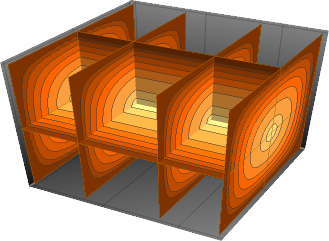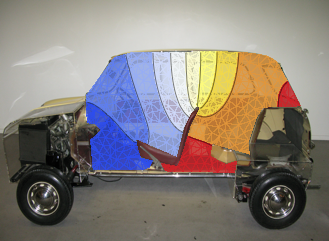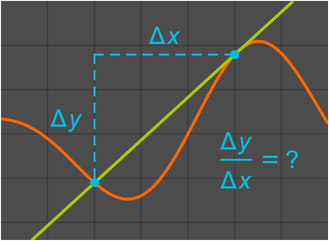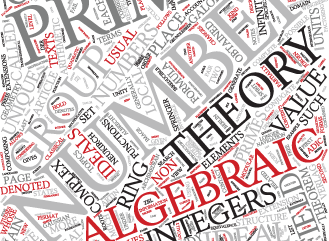# Mathematics

## Partial Differential Equations »Mathematica Version 11 adds extensive support for symbolic solutions of boundary value problems related to classical and modern PDEs. Numerical PDE-solving capabilities have been enhanced to include events, sensitivity computation, new types of boundary conditions, and better complex-valued PDE solutions. These developments provide powerful and flexible tools for modeling problems in physics, engineering, and other fields.

## Differential Eigensystems »Mathematica Version 11 extends its symbolic and numerical differential equation-solving capabilities to include finding eigenvalues and eigenfunctions over regions. Given a possibly coupled partial differential equation (PDE), a region specification, and, optionally, boundary conditions, the eigensolvers find corresponding eigenvalues and eigenfunctions of the PDE operator over the given domain. This functionality will enable you to gain a deeper understanding of your physics and engineering designs.

## Symbolic & Numeric Calculus »Mathematica Version 11 builds on its capabilities in calculus, with new symbolic and numeric features that enhance each other. Integral transforms are extended with the Mellin transform, along with related convolution and function simplification operations. The symbolic differential equation solver has been extended to support multiple types of integral and integro-differential equations. A new back end has been added to the numerical optimization engine for faster and more accurate solutions for large systems. Small tweaks include a new function for computing difference quotients and extending volume computations to understand non-Cartesian coordinates. Taken together, these enhancements make calculus faster, easier, and better at all levels of sophistication.